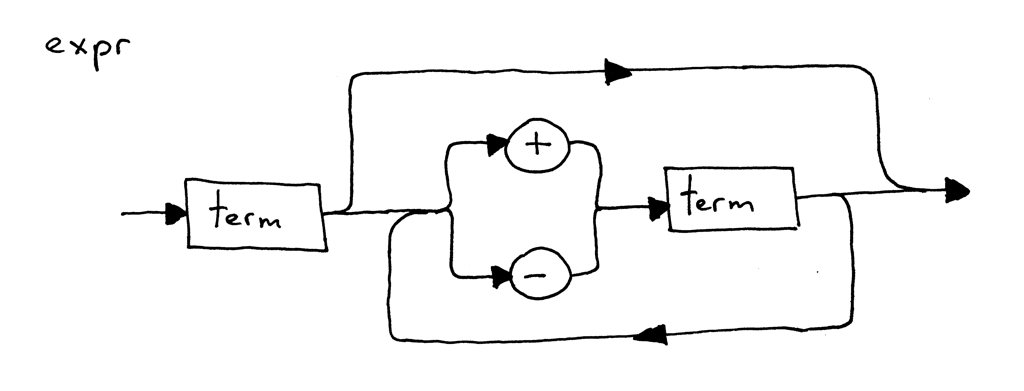# 来写一个简单的解释器（三）• 它们以图形方式表示编程语言规范（语法）。

• 它们可以帮助您编写解析器————您可以无脑的把图片内容“复制”到代码上。

• 3

• 3 + 4

• 7 - 3 + 2 - 1

  term = () => {
this.eat(INTEGER)
}

expr = () => {
this.currentToken = this.getNextToken()

this.term()
while ([PLUS, MINUS].indexOf(this.currentToken.type) !== -1) {
const token = this.currentToken
if (token.type === PLUS) {
this.eat(PLUS)
this.term()
} else if (token.type === MINUS) {
this.eat(MINUS)
this.term()
}
}
}


  term = (): number => {
const token = this.currentToken
this.eat(INTEGER)
}

expr = () => {
this.currentToken = this.getNextToken()

let result = this.term()
while ([PLUS, MINUS].indexOf(this.currentToken.type) !== -1) {
const token = this.currentToken
if (token.type === PLUS) {
this.eat(PLUS)
result += this.term()
} else if (token.type === MINUS) {
this.eat(MINUS)
result -= this.term()
}
}
return result
}


const INTEGER = 'INTEGER'
const PLUS = 'PLUS'
const MINUS = 'MINUS'
const EOF = 'EOF'

class Token {
type: string
value: any

constructor (type: string, value: any) {
this.type = type
this.value = value
}

toString = () => Token(${this.type},${this.value})
}

export class Interpreter {
text: string
pos: number
currentToken: Token = new Token(EOF, null)
currentChar: string | null

constructor (text: string) {
this.text = text
this.pos = 0
this.currentChar = this.text[this.pos]
}

error = (): never => {
throw Error('Error parsing input')
}

advance = () => {
this.pos++
if (this.pos > this.text.length - 1) {
this.currentChar = null
} else {
this.currentChar = this.text[this.pos]
}
}

skipWhitespace = () => {
while (this.currentChar != null && this.currentChar === ' ') {
}
}

integer = () => {
let result = ''
while (this.currentChar != null && /[0-9]/.test(this.currentChar)) {
result += this.currentChar
}
return Number(result)
}

getNextToken = (): Token => {
while (this.currentChar != null) {
if (/ /.test(this.currentChar)) {
this.skipWhitespace()
continue
}

if (/[0-9]/.test(this.currentChar)) {
return new Token(INTEGER, this.integer())
} else if (this.currentChar === '+') {
return new Token(PLUS, '+')
} else if (this.currentChar === '-') {
return new Token(MINUS, '-')
}
return this.error()
}
return new Token(EOF, null)
}

eat = (tokenType: string) => {
if (this.currentToken.type === tokenType) {
this.currentToken = this.getNextToken()
} else {
this.error()
}
}

term = (): number => {
const token = this.currentToken
this.eat(INTEGER)
}

expr = () => {
this.currentToken = this.getNextToken()

let result = this.term()
while ([PLUS, MINUS].indexOf(this.currentToken.type) !== -1) {
const token = this.currentToken
if (token.type === PLUS) {
this.eat(PLUS)
result += this.term()
} else if (token.type === MINUS) {
this.eat(MINUS)
result -= this.term()
}
}
return result
}
}


import { Interpreter } from '../src'

describe('Unit test', () => {
it('Interpreter run success', () => {
expect((new Interpreter('1+2')).expr()).toBe(3)
expect((new Interpreter('1+9')).expr()).toBe(10)
expect((new Interpreter('20+20')).expr()).toBe(40)
expect((new Interpreter('20-20')).expr()).toBe(0)
expect((new Interpreter('1-2')).expr()).toBe(-1)
expect((new Interpreter('1+2+3+4')).expr()).toBe(10)
expect((new Interpreter('10 + 1 + 2 - 3 + 4 + 6 - 15')).expr()).toBe(5)
})
})• 画一个语法图，仅包含乘法和除法表达式，比如“7 * 4 / 2 * 3”

• 支持仅包含乘法和除法的算术表达式，比如“7 * 4 / 2 * 3”

• 用其他语言写一个解释器，支持“7 - 3 + 2 - 1”这类的表达式。不要看本文章的例子。考虑一下它需要的东西：词法分析器（它接受把输入转化成Token流）、一个解析器（它从词法分析器提供的Token流中提取，并尝试理解其中的结构），解析器在成功解析后有解释器来解释算术表达式及其结果。将这些碎片串在一起。花一些时间将您获得的知识翻译成算术表达式的解释器吧！

• 什么是语法图？

• 什么是语法分析？

• 什么是语法分析器？